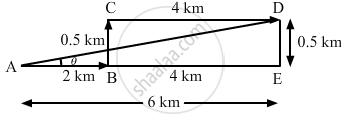Department of Pre-University Education, KarnatakaPUC Karnataka Science Class 11

# A Spy Report About a Suspected Car Reads as Follows. "The Car Moved 2.00 Km Towards East, Made a Perpendicular Left Turn, Ran for 500 M, Made a Perpendicular Right Turn, Ran for 4.00 Km and Stopped". - Physics

Derivation

A spy report about a suspected car reads as follows. "The car moved 2.00 km towards east, made a perpendicular left turn, ran for 500 m, made a perpendicular right turn, ran for 4.00 km and stopped". Find the displacement of the car.

#### Solution

The displacement of the car is represented by  $\overrightarrow{AD}$.

$\overrightarrow{AD} = 2 \hat {i}+ 0 . 5 \hat {j} + 4 \hat {i}$

$= 6 \hat {i} + 0 . 5 \hat {j}$

Magnitude of $\overrightarrow{AD}$ is given by

$AD = \sqrt{{AE}^2 + {DE}^2}$

$= \sqrt{6^2 + \left( 0 . 5 \right)^2}$

$= \sqrt{36 + 0 . 25} = 6 . 02 km$Now,

$\tan \theta = \frac{DE}{AE} = \frac{1}{12}$

$\Rightarrow \theta = \tan^{- 1} \left( \frac{1}{12} \right)$

Hence, the displacement of the car is 6.02 km along the direction $\tan^{- 1} \left( \frac{1}{12} \right)$  with positive the x-axis.

Concept: What is Physics?
Is there an error in this question or solution?

#### APPEARS IN

HC Verma Class 11, Class 12 Concepts of Physics Vol. 1
Chapter 2 Physics and Mathematics
Exercise | Q 7 | Page 29
Share